# 二叉堆

## 1 二叉堆的定义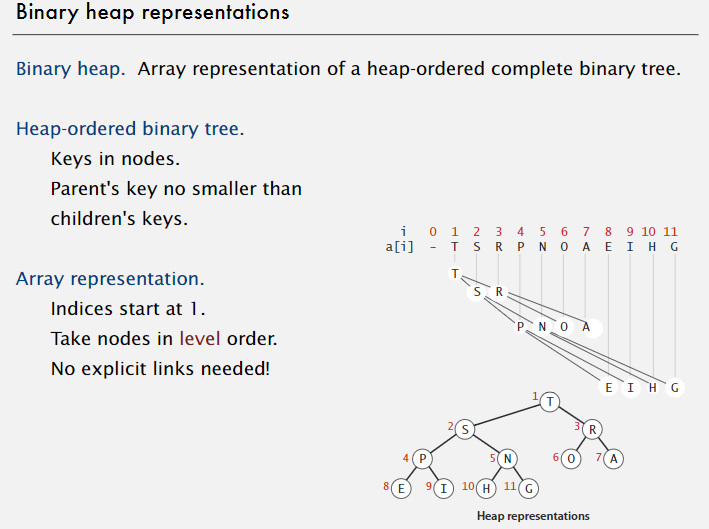## 2 二叉堆的性质

• 最大的元素在a (root结点)
• 每个k的父亲在k/2
• 每个k的孩子在k*2和k*2+1

## 3 二叉堆的操作

### 3.1 上浮（孩子大于父亲）——对应插入操作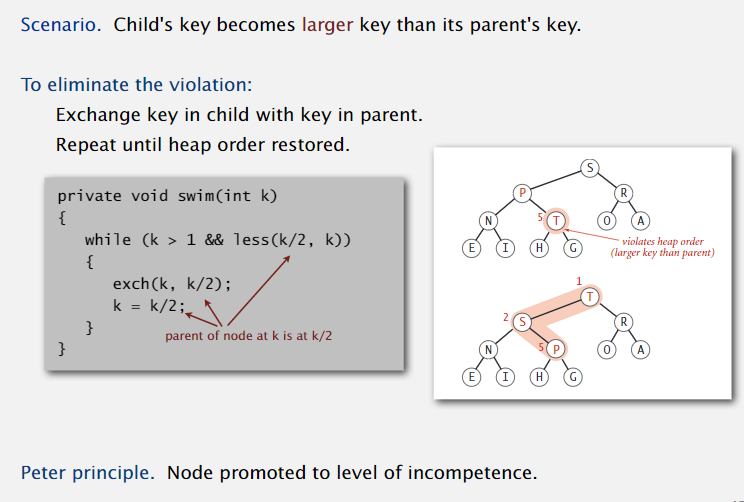### 3.2 插入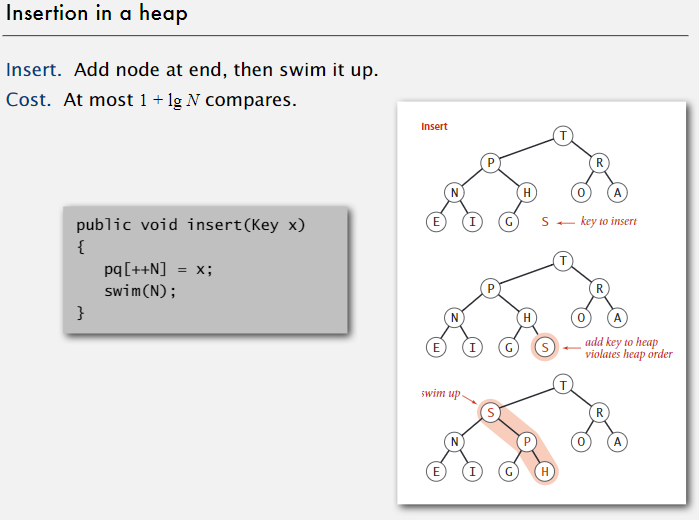### 3.3 下沉（父亲小于儿子）——对应删除操作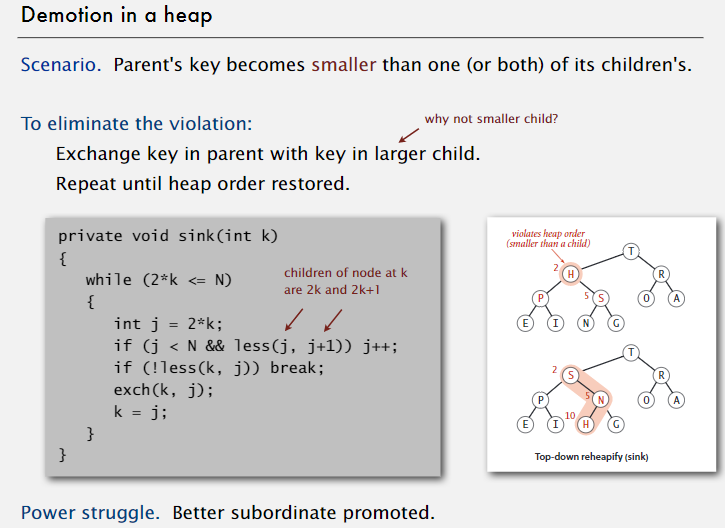### 3.4 删除最大元素## 4 二叉堆优先队列代码

/**
*
* @author rocky
*/
public class MaxPQ<Key extends Comparable<Key>> {

private Key[] pq;
private int N;

public MaxPQ(int capacity) {
pq = (Key[]) new Comparable[capacity + 1];
}

public boolean isEmpty() {
return N == 0;
}

public void insert(Key key)
{
N++;
pq[N] = key;
swim(N);
}

public Key delMax() {
Key max = pq;  //get the max element
exch(1, N);     //exchange between the root and the last element
N--;
sink(1);   //sink to the right place
pq[N+1] = null;   //delete
return max;
}

private void swim(int k)
{
while(k > 1 && less(k/2, k))
{
exch(k, k/2);
k /= 2;
}

}

private void sink(int k) {
while(k*2 <= N)    //if this node has left child
{
int child = k * 2;
if (child < N && less(child, child + 1)) {  //if the left child is less than the right child
child = child + 1;   //change child to the right child
}
if (less(k, child)) {
exch(k, child);
}
k = child;
}
}

private boolean less(int i, int j) {
return pq[i].compareTo(pq[j]) < 0;
}

private void exch(int i, int j) {
Key t = pq[i];
pq[i] = pq[j];
pq[j] = t;
}
}


# 5 二叉堆扩展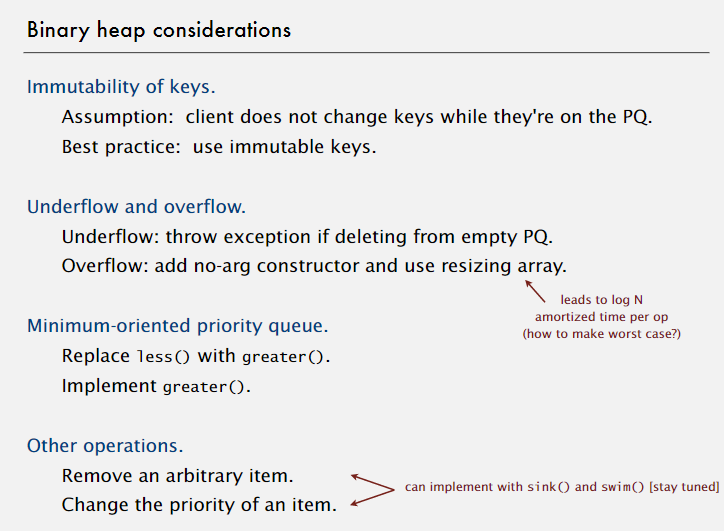## 5.1 不可变性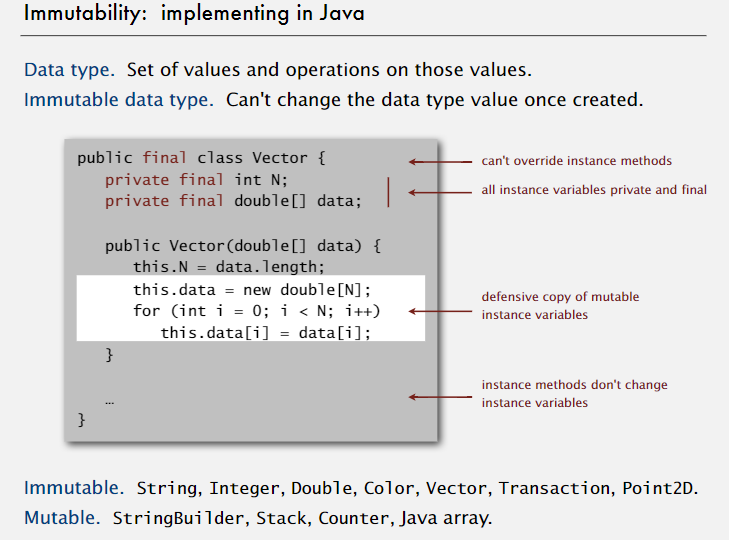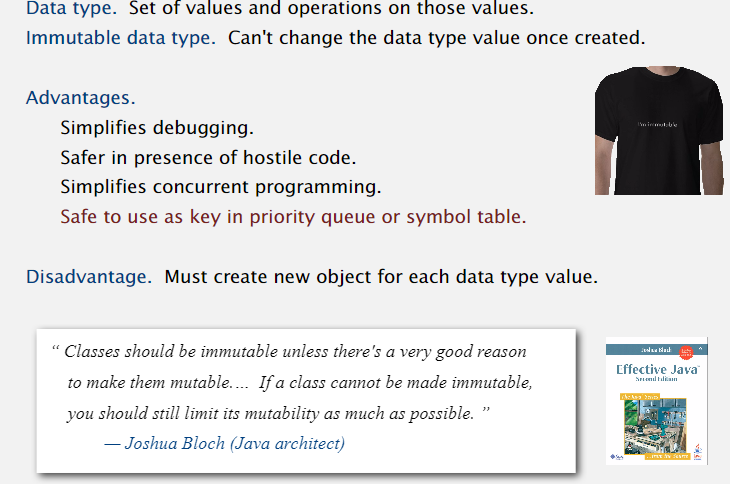posted @ 2016-04-28 21:27  voidsky  阅读(272)  评论(0编辑  收藏  举报